Along these lines,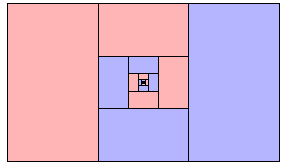13 + 19 + 127 + ⋅⋅⋅ = 12

one could get carried away. Here is one I like okay: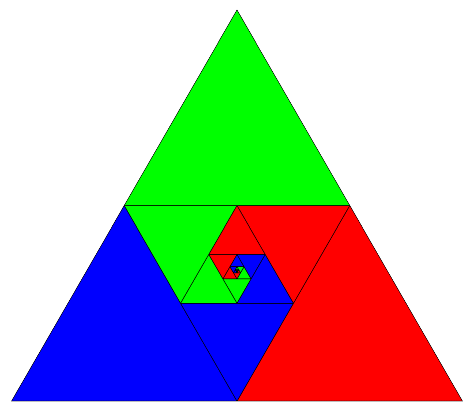14 + 142 + 143 + ⋅⋅⋅ = 13

And now I realize I have actually seen the following in the calculus text we use: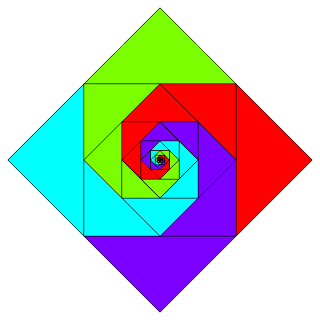18 + 116 + 132 + ⋅⋅⋅ = 14

(Of course, nobody needs much of a picture to understand the above equality.) How far can you go with this? I hoped to find something interesting by exploiting these analogs...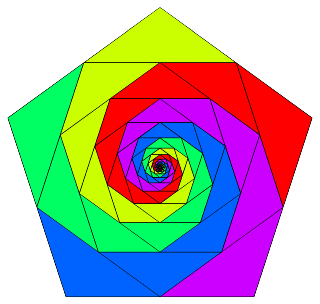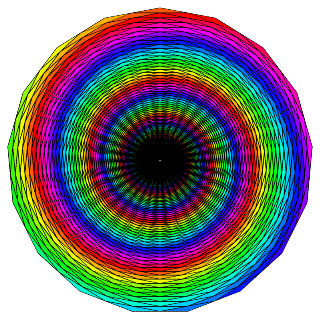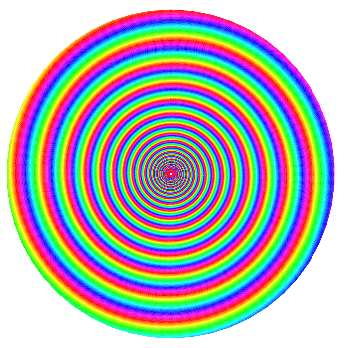but all I obtain from considering general N-gons is the rather lame identity that follows. (And it's derivation from the picture is hardly without need of explanation.)

 &infin∑ k = 0 (cos2 π/N)k = csc2 π/N

I suppose it is a decent exercise for a student to find the common ratio of triangle areas...

Rick

File translated from TEX by TTH, version 2.78, on 13 Feb 2001,
with subsequent HTML repair "by hand" of symbol fonts in 2009.